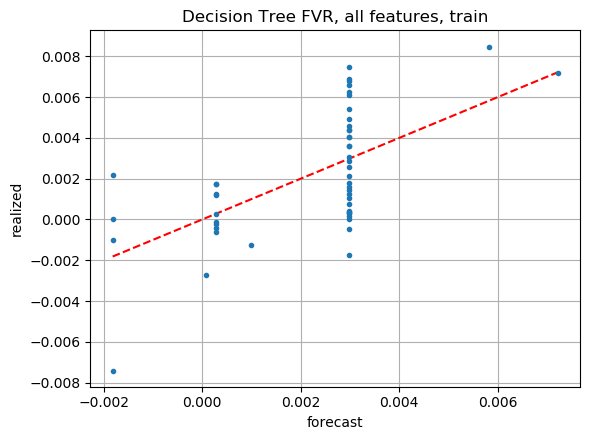# Decision tree regression

Continuing with baseline methods for comparison… Decision trees have been studied for decades, and innumerable enhancements have been proposed. Unfortunately, this complicates the situation.

## Hyperparameters

There are way too many hyperparameters, even in just the scikit-learn library version. I have no prior knowledge about which parameter values to use, so I have resorted to grid search with cross-validation. My first attempt ran all night without completing, despite the small size of my dataset. I had to reduce the number of options tested and run it on a bigger server, which still took hours. The subset of hyperparameters I varied included:

• criterion (mse, friedman_mse, mae)
• splitter (best, random)
• max_depth
• min_weight_fraction_leaf
• min_impurity_decrease

## Sample code

Here’s the relevant part of my python code for this post:

```from sklearn.tree import DecisionTreeRegressor
from sklearn.model_selection import GridSearchCV

model = DecisionTreeRegressor()

# create a dictionary of all hyperparameter values we want to test
param_grid = {}
param_grid['criterion'] = ["mse", "friedman_mse", "mae"]
param_grid['splitter'] = ["best", "random"]
param_grid['max_depth'] = np.arange(1, 5)
param_grid['min_weight_fraction_leaf'] = np.arange(0.02, 0.11, 0.02)
param_grid['min_impurity_decrease'] = np.logspace(-7, -5, num=3, base=10)

# use gridsearch to test all values for n_neighbors
model_gscv = GridSearchCV(model, param_grid, cv=4, n_jobs=8)

# fit model to data
model_gscv.fit(train_X, train_y, sample_weight=train_w)

print(model_gscv.best_params_)
# {'criterion': 'mse', 'max_depth': 3, 'min_impurity_decrease': 1e-07, 'min_weight_fraction_leaf': 0.02, 'splitter': 'random'}

train_pred = model_gscv.predict(train_X)
test_pred = model_gscv.predict(test_X)
```

## Dataset-1 model, all features

On dataset-1, the decision tree model with all features does not look ideal on the training data FVR plot:And the out-of-sample FVR plot looks indistinguishable from noise:

I don’t have interest in pursuing decision tree regression models any further. I much prefer my handcrafted model.